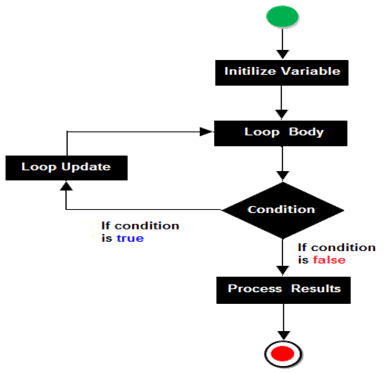# O Level Exam

#### do while Loop

The do while loop is an exit controlled loop, since the body of loop is executed first and then the test condition is evaluated. If it evaluates to false, then the loop is terminated, otherwise it is repeated. This means do while loop always executes at least once, even if the condition is evaluated to false.

Syntax:

`do{	statement 1;	statement 2;	…………….	statement n;}while(condition);`Differentiate between for loop and a while loop? What are it uses?

Answer: For executing a set of statements fixed number of times we use for loop while when the number of iterations to be performed is not known in advance we use while loop.

#### Programs

Write a C Program to Calculate the Sum of Natural Numbers

Write a C Program to Find Factorial of a Number

Write a C Program to Generate Multiplication Table

Write a C Program to Display Fibonacci Sequence

Write a C Program to Find GCD of two Numbers

Write a C Program to Find LCM of two Numbers

Write a C Program to Display Characters from A to Z Using Loop

Write a C Program to Count Number of Digits in an Integer

Write a C Program to Reverse a Number

Write a C Program to Calculate the Power of a Number

Write a C Program to Check Whether a Number is Palindrome or Not

Write a C Program to Check Whether a Number is Prime or Not

Write a C Program to Display Prime Numbers Between Two Intervals

Write a C Program to Check Armstrong Number

Write a C Program to Display Armstrong Number Between Two Intervals

Write a C Program to Display Factors of a Number

Write a program in C to print the sum of negative numbers, sum of positive odd numbers and sum of positive even numbers from a list of numbers entered by the user. The list terminates when the user enters a Zero.

Write program to generate multiplication table for first ‘n’ number, where ‘n’ is a user input.

Write a ‘C’ program to computer the average of every third integer lying between 1 and 200?

Write a program to check whether the given number is Perfect Number or not

Perfect Number :- Perfect number, a positive integer equal to the sum of its proper divisors. The smallest Perfect number is 6, which is the sum of 1, 2 and 3.

For Example:-

Input = 28

Output = 28 is the Perfect Number.

So as we can see that the divisor of 28 is 1, 2, 4, 7, 14, so the sum of all the divisors is 28 (1 + 2 + 4 + 7 + 14 = 28). Therefore, 28 is the Perfect number.